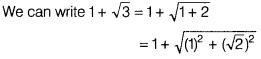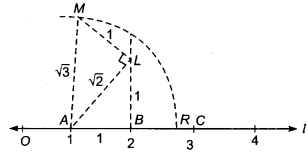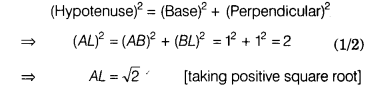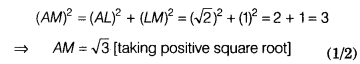# Represent (1 + √3) on the number line

Represent (1 + √3) on the number line.Draw a number line and mark all the points on it at an equal distance of 1 unit, such that
OA=AB=BC=1unit.Leave 1 unit (OA) and start from A, draw BL⊥l and cut-off BL = 1 unit.
Now, in ∆ ABL, by Pythagoras theorem,Again, at L,draw ML ⊥ AL and cut-off LM = 1 unit.
Now, in ∆ALM, by Pythagoras theorem, we haveNow, take A as centre and AM = √3 as a radius, draw an arc which intersects the number line at point R.
Hence, the point R represents 1 +√3.